# Triangles

What Thales realised was that he had created similar triangles with parallel lines.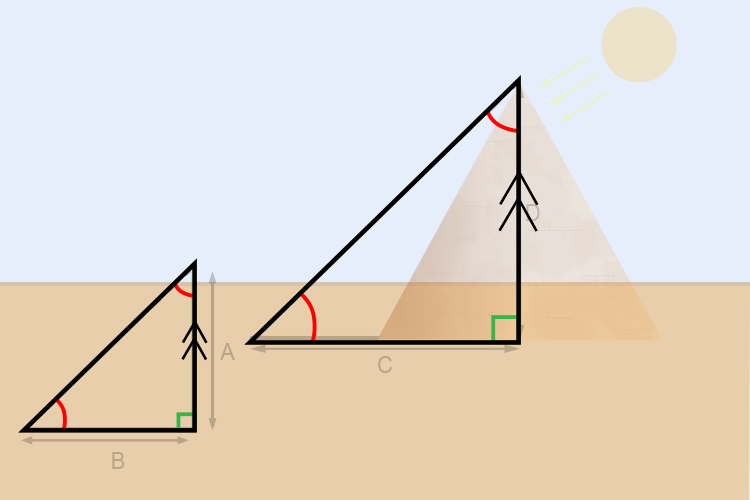As long as the two sides were parallel he could work out proportional values for the lengths of the lines.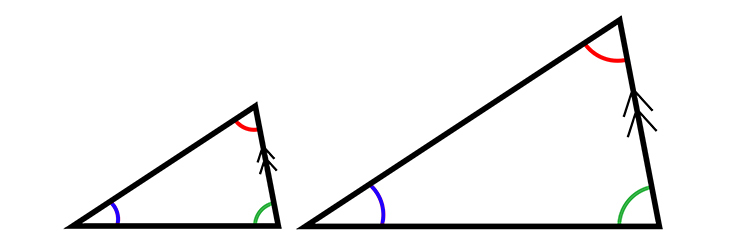Similar triangles with two parallel lines.

So Thales then used this information to measure the width of a river.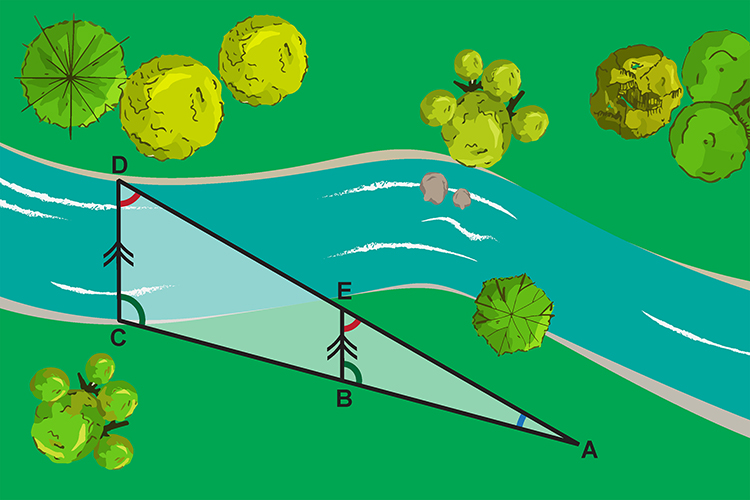As long as CD and BE are parallel he found the width of this river because of similar triangles with parallel lines.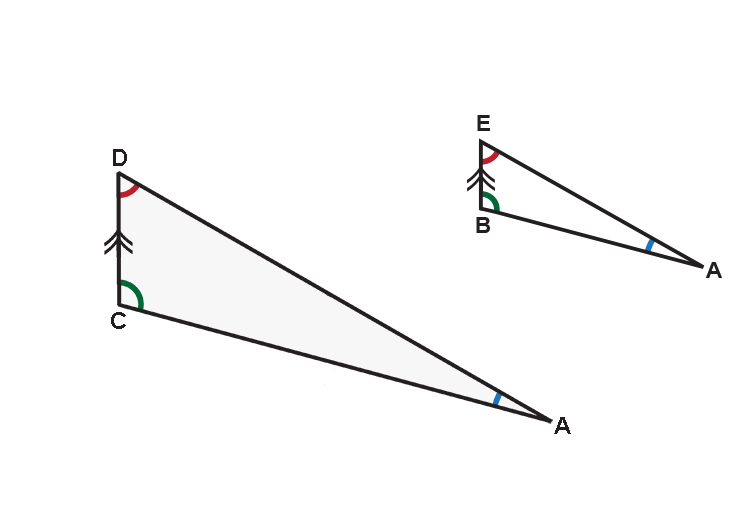Similar triangles with parallel lines.

And therefore he found the ratio

(CD)/(AC) is identical to (BE)/(AB)

Thales could measure A to C and A to B and B to E and so he could determine C to D i.e. distance across the water.

Thales extended this to working out how far away ships were directly from his line of vision.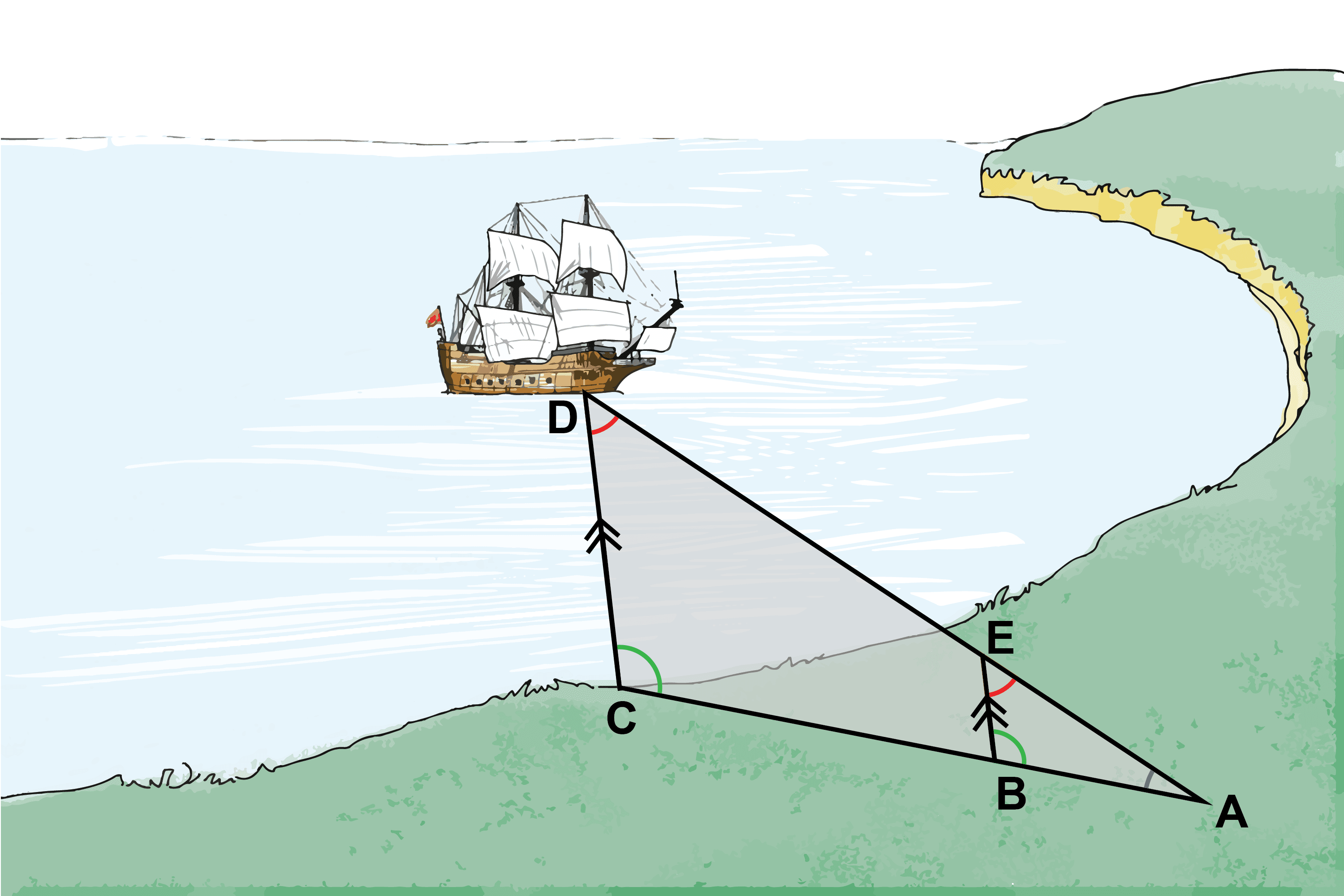Even if the ship was miles out he could determine how far away the ship was. His method could even be used for working out firing distances.

He found

(A\ text(to)\  B)/(A\ text(to)\  C)=(A\ text(to)\  E)/(A\ text(to)\ D)

He then realised that as long as the triangles were similar EVERYTHING IS PROPORTIONAL to each other as ratios.

Even (A\ text(to)\  B)/(A\ text(to)\  C)=(B\ text(to)\E)/(C\ text(to)\D)

Or (A\ text(to)\  E)/(A\ text(to)\  D)=(B\ text(to)\E)/(C\ text(to)\D)

Conclusion

Thales' Intercept theorem – If two intersecting lines are intercepted by a pair of parallel lines, then the segments created are proportional.

So, in this example: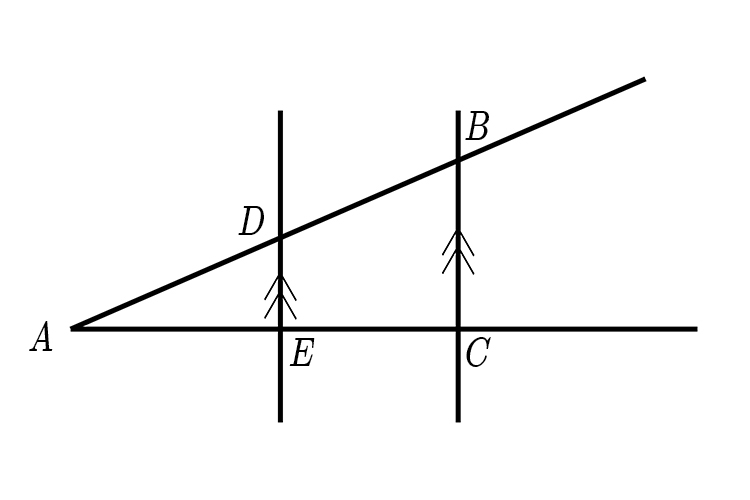(AE)/(AC)=(AD)/(AB)=(ED)/(CB)